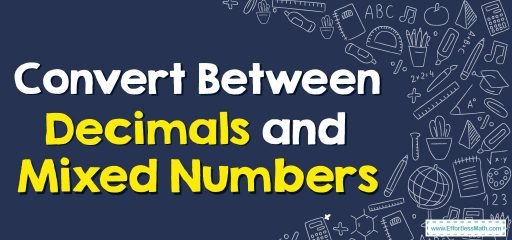# Convert Between Decimals and Mixed NumbersNumbers on the left-hand side of the decimal point show there’s a whole part and several pieces of a whole.

Decimal numbers have two parts that are separated by a decimal point:

Whole part: The numbers on the left-hand side of a decimal point.

Decimal or the fraction part: The numbers on the right-hand side of a decimal point.

To convert a decimal number into a mixed number, the whole number is kept the same and the decimal part is converted.

### Convert Between Decimals and Mixed Numbers – Examples 1

Write 3.6 as a mixed fraction.
Solution:
Step 1: Add 1 to the denominator of the decimal number (3.6).
Step 2: Multiply its denominator and the numerator by multiples of 10.
$$3.6=\frac{3.6}{1}=\frac{3.6}{1} \frac{×10}{×10}=\frac{36}{10}$$

### Convert Between Decimals and Mixed Numbers – Examples 2

Write $$6 \frac{1}{2}$$ as a decimal number.
Solution:
Multiply the numerator and denominator until the denominator becomes a power of 10.
The numbers to the left of the decimal point in the mixed numbers are the integers.
The decimal number to the right of the decimal point in the mixed numbers is the fractional number.
$$6 \frac{1}{2}=6 \frac{1}{2} \frac{×50}{×50}=6 \frac{50}{100}=6.5$$

### What people say about "Convert Between Decimals and Mixed Numbers - Effortless Math: We Help Students Learn to LOVE Mathematics"?

No one replied yet.

X
30% OFF

Limited time only!

Save Over 30%

SAVE $5 It was$16.99 now it is \$11.99